Module 2.1 Notes "Present and Describe Linear Relationships"

 Index to Module Two Notes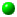2.1: Present and Describe Linear Relationships2.2: Test Linear Relationships and Make Predictions

Regression analysis involves the study of the form and direction of the relationship between two or more variables. The main purpose of regression analysis is to predict the value of a dependent or response variable based on values of the independent or explanatory variables. Simple linear regression analysis involves the study of the linear or straight-line relationship between two numerical variables: the dependent variable and one numerical explanatory variable.

Correlation analysis involves the study of the strength of the relationship between two variables. A supporting role of correlation analysis is to discover those explanatory variables that are strongly related to the response variable to improve the predictions made. For example, suppose we want to predict the number of hours it would take to perform an audit on a client. One explanatory variable might be be the dollar amount of client assets. Another explanatory variable might be the number of employees. After gathering and analyzing data we discover that the correlation between hours and assets is much higher than between hours and employees. In this case, we would be better off using assets as the explanatory variable.

This set of module notes introduces techniques for presenting and describing simple linear regressions and correlations. Module 2.2 Notes describe how we test linear regressions for statistical significance and practical utility, and how the linear regression model can be used for prediction. The outline of steps to conduct a complete simple linear regression and correlation analysis is:

1. Hypothesize the regression model relating the dependent and independent variables.
2. Gather data and describe the form and direction of the relationship with a scatter diagram.
3. Estimate the regression model parameters and the correlation coefficient.
4. Test the practical utility of the regression model.
5. Test the statistical utility of the regression model.
6. Evaluate the assumptions of the regression model.
7. Use model for prediction.

This set of module notes will carry us through Steps 1 through 3 above. Module 2.2 Notes will cover Steps 4 through 7. In Module 3 we will expand this model to consider the relationship between the dependent variables and multiple independent variables, including nonlinear terms and categorical variables.

Step 1: Hypothesize the Regression Model Relating the Dependent and Independent Variables

The dependent or response variable, identified by the symbol Y, is the variable we wish to predict. The independent or explanatory variable, identified by the symbol X, is the predictor variable. In simple linear regression, we propose the following population straight-line model relating Y and X:

Eq. 2.1.1: Y = B0 + B1X + e, where:
Y = Dependent Variable
X = Independent Variable
B0 = Y Intercept=mathematical value of Y when X=0
note: Unless there are X values of 0, the Y intercept has no practical interpretation, just a mathematical interpretation as we will see later with an example.

B1 = Slope = the amount of increase in Y (or decrease if

B1 has a negative sign) when X increases one unit .

e = Random error

For a particular observation, for example the "ith" observation, this equation becomes:

Eq. 2.1.2: Yi = B0 + B1Xi + ei

This equation implies that each observation in a set of data has an actual Y value, an X value, a predicted Y value, and error which is the actual Y value minus the predicted Y value. In regression analysis, one of our objectives is to select those predictor variables that result in as little error as possible, recognizing there will always be some error in prediction. This equation is often referred to as the probabilistic model relating Y to X. The deterministic model is just the straight-line or prediction part without the actual value of Y and its error:

Eq. 2.1.3: E(Y) = B0 + B1X, where
E(Y) = Expected Value of Y

In step two, we will fit a straight-line model based on sample data to estimate the above simple linear regression equation.

That's enough theory. Let's go to step two, look at some data, and create the scatter diagram.

Step 2: Gather Data and Describe the Form and Direction of the Relationship with a Scatter Diagram

The example to illustrate simple linear regression analysis is about a audit company - that is, a company that is in the business of performing financial audits. This company maintains a very small internal workforce and thus relies of external auditors to perform client audits. The company would like a model to predict the number of external audit hours it would need to contract in order to do an audit. Such a model would be very helpful in budgeting and planning. Management believes that a good predictor variable would be client assets. In order to build the model, a sample of data must be gathered.

Worksheet 2.1.1 shows the result of the sample. The first column, Assets, are values of the independent variable (this is the X variable) in thousands of dollars. The second column, ExtHours, contains values of the dependent variable (this is the Y variable) in hours. So, the first row of numbers represents an audit completed in the past for a client with assets of \$ 3,200,000. The audit company had to contract for 700 external hours to perform the audit. Note that in regression analysis, every observation has two values, an X value and a Y value.

Worksheet 2.1.1

 Assets ExtHours 3200 700 3000 900 3500 800 4000 900 4700 880 5000 850 6000 1000 5500 1000 6500 950 6500 1100 7000 1200 4500 830 7500 1100 5750 1100 7250 1160 8000 1120 8000 1300 8500 1400 9000 1500 8500 1200

In the Assignment section of the Main Module 2 web page, you will see that the first item for Assignment 2 is entering the X and Y data in an Excel Spreadsheet. Hopefully, you can think of a good response variable from your work , service or home environment. Perhaps you would like to predict profit contribution, sales, or salary, or hours to complete a task. Once you determine what you would like to predict or understand, then pick a variable that you think explains or predicts your response variable. Perhaps labor cost is a good X (cost driver) to predict profit contribution (Y). Perhaps years of experience is a good X variable to predict salary (Y). Once you select your X and Y variables, try to collect 50 observations. In regression and correlation analysis, an observation involves an X and a Y value. For example, sales in month 1 were 334 units. Here, 1 is the value for X and 334 is the value for Y for the first observation. Another example, an employee in the database earns \$50,000 (the value of Y for this sample observation) and has worked for 22 years (the value of X for this sample observation). Fifty observations is more than the minimum required, so you can get by with less if you have to. The minimum required for a two-variable regression model is 20 observations (10 observations per variable). The next task is to create the scatter diagram.

In regression analysis, the scatter diagram is used to plot the independent variable on the X or horizontal axis, and the dependent variable on the Y or vertical axis. To produce a scatter diagram, highlight the X and Y data columns including the column titles. Then select the Chart Wizard on the Standard Toolbar, then XY Scatter, then respond to the dialog screen questions. It will take a couple of tries to get the hang of making scatter diagrams; but after some practice you should be able to replicate the scatter diagram shown in Worksheet 2.1.2. In Assignment 2, the second item is for you to create a scatter diagram.

Worksheet 2.1.2.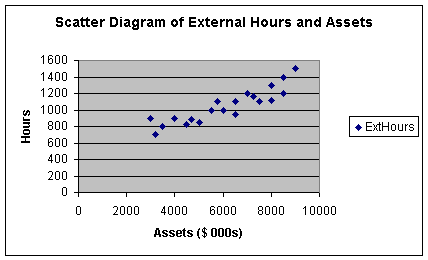Note that as I was going through the dialogue boxes, I used the opportunity to label the X and Y axis's, as well as give the diagram a title. This scatter diagram shows a positive form of relationship between X and Y, meaning that when X increases, Y increases. It appears that when X increases, Y increases at a constant rate, meaning that the form of the relationship is linear.

A comment on page presentation. If you click on File on the Standard Toolbar, then Print Preview, you can see where the scatter diagram will appear on the worksheet page. If you want to move it, just click on any part of the white area of the diagram and click and drag the chart. If you want to change the shape of the chart, click on the chart again and note the squares along the borders of the chart. If you click and drag on the middle squares you can make the chart wider, narrower, longer or shorter. Note finally that when you click on any chart, the word Data changes to Chart on the Standard Toolbar so you can switch between data functions and chart functions.

Let's summarize what we have learned thus far. Regression analysis includes the study of the form and direction of the relationship between dependent and independent variables. In this case, we have one dependent (Y) and one independent variable (X). The form of a relationship can be linear or curvilinear. The form in Worksheet 2.1.2. above happens to look like a linear relationship. Worksheet 2.1.3 illustrates a curvilinear relationship.

Worksheet 2.1.3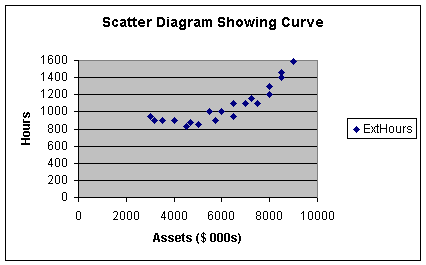Note with the curvilinear relationship, as assets increased initially, external audit hours remained relatively constant up to clients with assets of approximately \$5,000,000. Then it appears that external hours increase at a slightly increasing rate from \$5,000,000 to \$9,000,000. We will see in Module 3 that this is curvature: Y increases at an increasing rate as X increases. Curvature also occurs when Y increases at a decreasing rate as X increases.

Before continuing with the example, let's summarize the direction component of the relationship. Our example in Worksheet 2.1.2 shows a positive direction. Worksheet 2.1.4 shows what a negative direction would look like.

Worksheet 2.1.4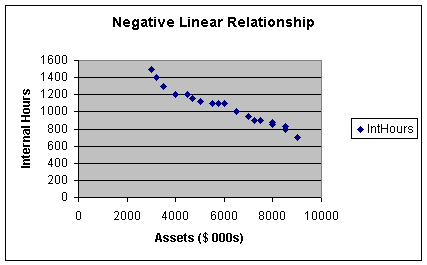In this worksheet, as assets increase, internal hours decrease: this describes a negative relationship between X and Y.

Pause and Reflect

To describe the relationship between two variables, we look at the form (linear or curvilinear) and the direction (positive or negative) of the relationship. Linear form means that as X increases, Y increases or decreases at a constant rate. Positive direction means that Y increases when X increases; and negative direction means that Y decreases when X increases.

The last component of the relationship between two variables is strength. We will talk about measuring strength in Step 3, as we need some numbers to do that.

Step 3: Determine the Simple Linear Regression Equation and Correlation Coefficient

Regression Coefficients
Our next step is to find values for b0 and b1 in the following simple linear regression equation:

Eq. 2.1.4: y = b0 + b1x

This equation, based on sample data, is used to estimate the hypothesized population Eq. 2.1.3. Note I have made all of the symbols lower case to distinguish the sample equation from the population equations shown as Eq. 2.1.1. - Eq. 2.1.3. Some texts put hats ( ^ ) on the symbols in Eq. 2.1.4 to distinguish the sample equation from the population model. Our task is to estimate numerical values for the intercept, b0, and the slope, b1. These are called the regression parameters in the simple linear regression equation (the equation is also known as the least squares regression equation or the trend equation or simply the regression).

If you were a careful artist, you could take a ruler and draw a straight-line as close as possible to every point in Worksheet 2.1.2. Then, extend the left end of that line to the Y axis. The y value at the point where an extension of the line touches the Y axis is called the intercept, the value of y when x equals zero. Next, anywhere on the line, draw a horizontal line one unit long in the X direction. Now draw a vertical line to the regression equation. The length of the vertical line divided by the length of the horizontal line represents the amount of change in Y for the unit change in X. This is called the slope of the line. Don't be alarmed - we will let the computer do the "line drawing" to estimate the slope and the intercept - I just wanted to go over the concept.

Actually, the computer uses mathematics to solve equations to determine the value of the slope and intercept. The technique is called the least squares method of regression. It essentially involves trying to minimize the error (actual value of Y minus the predicted value of y) in the equation Sum (Y - y)2. To let Excel do the work, first make a copy of the scatter diagram to preserve the original. To copy the diagram, put the cursor anywhere in the white area of the the scatter diagram chart. When you click the left mouse button, the chart becomes highlighted (small squares or handles appear around the border of the chart). Now select Edit on the Standard Toolbar and Copy from the pulldown menu. Now move the cursor, select a new cell of the worksheet, and select Edit on the Standard Toolbar and Paste from the pulldown menu. You should get another copy of the scatter diagram.

Now select (highlight) the copy of the scatter diagram by clicking anywhere on the white chart surface and select Chart on the top menu bar. Note that this menu bar has the word Data instead of the word Chart unless you have highlighted a chart, such as the scatter diagram. Next select Add Trendline from the pulldown menu and you will get a dialog box. The default Linear trend/regression is what we want. Before selecting OK, select the Options Tab. Then select Display Equation and Display R-Square. You should get Worksheet 2.1.5, as shown below.

Worksheet 2.1.5The least squares regression equation, or simply, the linear regression equation, is shown as:

Eq. 2.1.5: y = 440.05 + 0.1x, since y is ExtHours and x is Assets,
ExtHours = 440.05 + 0.1(Assets).

After we finish Steps 1- 6, we will use this equation to make a prediction. To jump ahead, what if we want to predict the hours it will take to audit a company with \$6,000,000 in assets. Looking at the Worksheet 2.1.5 regression line, if we go straight up from 6000 on the X axis, we touch the line at a y value a little over 1,000 hours. To be more accurate, we can substitute 6000 into Eq. 2.1.5 and get:

Eq. 2.1.6: y = 440.05 + 0.1 (6000) = 440.05 + 600 = 1040.05.

Note carefully that I substituted 6000 into Eq. 2.1.6 rather than 6000000 since the original data was entered in thousands.

However, before we use the equation for prediction we have to test it's practical and statistical utility (Steps 4 and 5). For now, let's be sure we understand how to interpret the equation. The intercept is 440.05. This means that the value of y (External Hours) is 440.05 when x (assets) equals zero. Now this is really just a theoretical point helpful in placing the equation on the scatter diagram. It is theoretical without practical value because we did not have any x (asset) values equal to zero in the original data. Some suggest that the intercept is like a fixed value - what we need to get started without any value for x at all. But to know this, we would have had to include observations where x in fact equals zero. Otherwise, we are just guessing. In fact, an ethical caution in regression is not to interpret the results of regression models outside of the range of the original data.

Now let's look at the slope, which is 0.1. The slope is interpreted as follows: y (External Hours) is predicted to increase 0.1 when x (assets) increase by one. To make this a bit more practical, we can say that External Hours increase by 0.1 when Assets increase by \$1,000 (since our data was is in thousands of dollars, 1 unit of x is equal to \$1,000). Since the relationship is linear and the coefficients are proportional, we can also say that external hours increase by 1 when assets increase by \$10,000; or external hours increase by 10 when assets increase by \$100,000; or external hours increase by 100 when assets increase by \$1,000,000. Now we have something! The firm should plan on 100 more external hours for every increase of \$1,000,000. Caution as before: this interpretation only applies within the range of our data. We don't know what the slope is above \$9,000,000 since we did not have any observations above \$9,000,000. We do not extrapolate beyond the range of our data when making interpretations.

Pause and Reflect

The intercept in the regression equation is the value of y when x equals zero. It has no practical interpretation unless the regression model was built on data where some of the values of x were zero. The slope of the regression equation indicates the predicted change in y (increase if the slope is positive; decrease if the slope is negative) for a one-unit increase in x.

Regression equations are the most widely used statistical tools in business since they can be used to predict the value of a response variable, such as sales, based on a predictor variable. We discussed form and direction as important aspects of the relationship between the two variables. The strength of the relationship between two variables is also an important aspect to know about in business.

Correlation Analysis
Recall earlier that we said correlation analysis is used to measure the strength of the linear relationship between two quantitative variables. To find the correlation coefficient, we begin with the coefficient of determination, R2.

Look back at Worksheet 2.1.5 and note the R2 = 0.8173 or 0.82 on the scatter diagram. R-Square, or R2, is the symbol for the coefficient of determination. We will see its math later. For now, the interpretation of R2 is simply the amount of sample variation in Y that is explained by X. For my example, we would say that client assets explain 82% of the sample variation in external hours.

As you look at a scatter diagram you notice that the value of Y changes or varies for different values of X. Strongly related variables are those in which changes in X result in predictable changes in Y. In other words, X is explaining a large percent of the variation in Y. Weakly related variables, such as those with R2 below 25%, suggest that changes in X do not result in predictable changes in Y. We will have more to say about R2 when we get to Step 4 in Module 2.2 Notes. I'll close this brief introduction with the note that R2 should be as close to 100% as possible in order for us to have models that are practically useful. A good general benchmark is that R2 should be at least above 50%, although it should be noted that specific industries/service sectors may have their own traditional benchmarks for R2.

The correlation coefficient, r, is the statistic commonly used to report the strength of a linear relationship between two variables. In fact, the word has crept into common English usage when we say something like, "there is a high correlation between how much I study and my GPA" (at least I hope we say something like that!). The correlation coefficient is simply the square root of R2. For this example, r = +0.904.

This r of +0.904 represents a strong, positive, linear relationship between client assets and external hours. How do I get the direction? By looking at the sign on the slope coefficient. If the sign is positive, r is positive, and vice-versa. Worksheet 2.1.4 shows a relationship in which the r would have a negative sign. How do I get the measure of strength? That one is tougher but here are some benchmarks that are common in general business/service sectors (you may find different benchmarks in medical practice, psychology, and specific industries/service sectors, and so forth):

r = -0.9 (and below): Strong negative linear relationship
-0.7: Moderate negative linear relationship
-0.5: Weak negative linear relationship
+0.0: No relationship
+0.5: Weak positive linear relationship
+0.7: Moderate positive linear relationship
+0.9 (and above): Strong positive linear relationship

There are two cautions with using the correlation coefficient. First, we can say that X and Y are strongly related, which implies that changes in X result in predictable changes in Y. But unless we do an experiment, we are cautioned against saying that X causes Y from an ethical perspective. Think about examples of this. The r between consumption of the alcohol beverage Scotch and donations to charitable organizations is very high, such as above a positive 0.90. We would not say that such consumption causes donations to increase, or reduced consumption causes donations to decrease because the causation variable is probably disposable personal income. When DPI goes up, donations and consumption go up. That being said, we can still rely on the value of r to select variables that have an impact or result in a change in Y, without having to do an experiment. That is, marketing executives in the Scotch industry can still pattern sales projections off projections of aggregate donations to charitable organizations - to make predictions, you do not have to prove causation.

The second caution is to remember that r explains the strength of linear relationships. Look at the following example in Worksheet 2.1.6.

Worksheet 2.1.6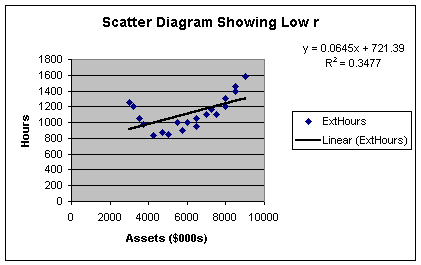The R2 here is only 35%; meaning that client assets now only explain 35% of the sample variation in external hours. This gives an r of +0.59, which borders on a weak relationship. In actuality, the relationship between client assets and external hours is indeed strong - but the strength lies in the curvilinear relationship between the two variables, not the linear relationship. More on that in Module 3. For now, just recognize that many people misapply the correlation coefficient to models that have curvilinear rather than linear form.

A closing comment on correlation analysis. Since r is dimensionless and varies between -1 and +1, it can be thought of as a standardized measure of the strength of the linear relationship between two variables. Related to the correlation coefficient is covariance, a non-standardized measure of the strength of the linear relationship between two variables. The covariance is computed by multiplying the correlation coefficient by the product of the standard deviations of the two variables, thus mathematically defining the relationship. While the correlation coefficient is the more commonly used measure of the strength of the linear relationship between two variables, financial models such as used in portfolio theory incorporate covariance so you may see that statistic in a finance class.

Pause and Reflect

Steps 1 - 3 of regression and correlation analysis give us information about the form, direction and strength of the relationship between two variables. In simple linear regression and correlation analysis, it is assumed that the two variables are numerical and that the form of the relationship is a straight-line. While these may seem simplistic assumptions, many relationships in business and economics are modeled in this fashion.

This closes Module 2.1 Notes. You should be able to get through Items 1 through 4 of Assignment 2 at this point.

Outliers and Influential Variables
Before we go to Module Notes 2.2, let me illustrate one last caution in Steps 1 - 3 that you may run into as you prepare for Assignment 2. Recall that we relied on the histogram in Module 1 to identify outliers to the distribution under examination. We can also have outliers in regression analysis. Let's look at a modified scatter diagram in Worksheet 2.1.7.

Worksheet 2.1.7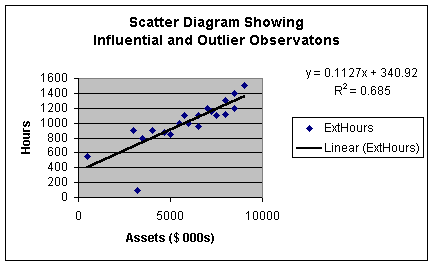This scatter diagram is similar to that in Worksheets 2.1.2 and 2.1.5 except that I changed the value of two of the observations. The observation with assets of just over \$3,000,000 and external hours of 100 is well below the regression line. This would lead us to expect that it is an outlier to the regression model. When we get to Module Notes 2.2, we will look at a way to precisely determine if that observation is an outlier or not. We use the same rules as before - if an observation is more than 3 standard deviations from the regression line, it is an outlier.

There is one other observation that appears apart from the data. It is the observation with a value of fewer than 600 external hours and less than \$1,000,000 in assets. While this observation is separated from the data, it is quite close to the regression line. Thus, it is not an outlier to the regression model. However, since the point is separated from the data, we call it an influential observation. As in our study of descriptive statistics for individual variables in Module 1, outliers and influential variables should be identified and removed from the data set prior to numerical analysis. As before, sometimes outliers and influential observations suggest a need to stratify the data before further analysis; sometimes outliers and influential observations are just individual events (sometimes even input errors!) that should be removed before further analysis.

Reference:

Anderson, D., Sweeney, D., & Williams, T. (2001). Contemporary Business Statistics with Microsoft Excel. Cincinnati, OH: South-Western, Chapter 3 (Section 3.1) and Chapter 12 (through Section 12.8).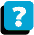About the Course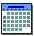Module Schedule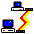WebBoard